# Orthogonal system

An orthogonal system of vectors is a setof non-zero vectors of a Euclidean (Hilbert) space with a scalar productsuch that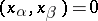when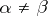. If under these conditions the norm of each vector is equal to one, then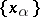is said to be an orthonormal system. A complete orthogonal (orthonormal) system of vectorsis called an orthogonal (orthonormal) basis.

M.I. Voitsekhovskii

An orthogonal coordinate system is a coordinate system in which the coordinate lines (or surfaces) intersect at right angles. Orthogonal coordinate systems exist in any Euclidean space, but, generally speaking, do not exist in an arbitrary space. In a two-dimensional smooth affine space, orthogonal systems can always be introduced at least in a sufficiently small neighbourhood of every point. It is sometimes possible to introduce orthogonal coordinate systems in the large. In an orthogonal system, the metric tensor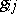is diagonal; the diagonal componentsare called Lamé coefficients. The Lamé coefficients of an orthogonal system in space are expressed by the formulas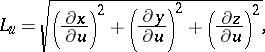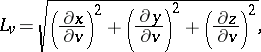where,andare Cartesian coordinates. The Lamé coefficients are also used to express the line element: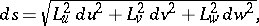the element of surface area:the volume element: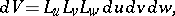and the operations of vector analysis: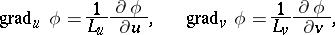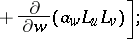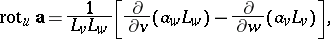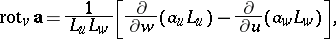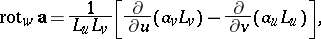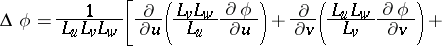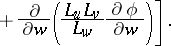The most frequently used orthogonal coordinate systems are: on a plane — Cartesian coordinates; elliptic coordinates; parabolic coordinates; and polar coordinates; in space — cylinder coordinates; bicylindrical coordinates; bipolar coordinates; paraboloidal coordinates; and spherical coordinates.

D.D. Sokolov

An orthogonal system of functions is a finite or countable system of functions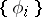belonging to a space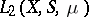and satisfying the condition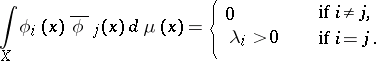If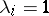for all, then the system is orthonormal. It is supposed that the measuredefined on the-algebraof subsets of the setis countably additive, complete, and has a countable base. This definition encompasses all orthogonal systems studied in analysis. Such systems are obtained for various concrete realizations of the measure space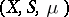.

The greatest interest is in complete orthonormal systems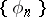, which possess the property that for any functionthere is a unique series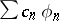which converges toin the metric of the space. The coefficients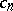are defined by the Fourier formula:These systems exist by virtue of the separability of the space. A universal method of constructing complete orthonormal systems is given by the Gram–Schmidt orthogonalization method. This method can be applied to any complete linearly independent sequence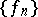of functions in.

Important examples of orthogonal series are obtained by considering the space(in this case,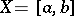,is the system of Lebesgue-measurable sets andis the Lebesgue measure). Many theorems on the convergence or summability of a serieswith respect to a general orthogonal systemin the space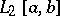are also valid for series with respect to orthonormal systems in the space. Moreover, in this particular case, interesting concrete orthogonal systems have been constructed which possess some nice properties. These systems include the Haar, Rademacher, Walsh–Paley, and Franklin systems.

a) The Haar system: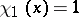,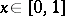,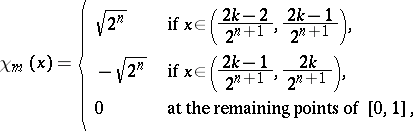where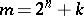,,. Series with respect to the Haar system are typical examples of martingales (cf. Martingale) and thus the general theorems of martingale theory are also correct for them. Moreover, the systemis a basis in,, and the Fourier series with respect to the Haar system of any integrable function converges almost-everywhere.

b) The Rademacher system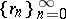: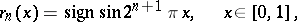is an important example of a stochastically-independent orthogonal system of functions and is used both in probability theory and in the theory of orthogonal and general series of functions.

c) The Walsh–Paley system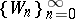is defined using the Rademacher functions: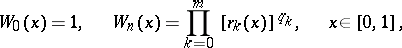where the numbersandare defined using the binary expansion of the number: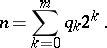d) The Franklin system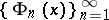is obtained by Gram–Schmidt orthogonalization of the sequence of functionsIt is an example of an orthogonal basis of the space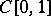of continuous functions.

In the theory of multiple orthogonal series, function systems of the form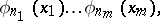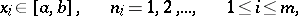are examined, whereis an orthonormal system in. These systems are orthonormal on the-dimensional cube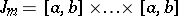, and are complete if the systemis complete.